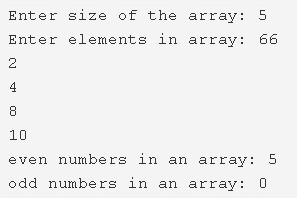# C Program To Count Number Of Even & Odd Elements In Array | C Programs

C program to count the total number of even and odd elements in an array – In this article, we will brief in on the numerous methods to count the total number of even and odd elements in an array in C programming.

Suitable examples and sample programs have also been added so that you can understand the whole thing very clearly. The compiler has also been added with which you can execute it yourself.

The methods used in this piece are as follows:

• Using Standard Method
• Using Function

An array, as we all know, is a collection of elements in a horizontal fashion which are denoted with the help of specific location based parameters known as pointers. Arrays form an integral part of C programming.As you can see in the example specified above, you need to enter the size of the array first.

The size of the array given is 5.

Then, you have to enter the elements in any particular order of your choice.

The elements entered here are as follows:

66 2 4 8 10

So, we can see that there are a bunch of numbers.

Even numbers: Numbers which are perfectly divisible by 2.

Odd numbers: Numbers which leave behind a remainder of 1 when divided by 2.

Hence, it is seen that there are 5 even numbers and 0 odd numbers.

Thus, the methods to count the same in C programming are as follows:

## Using Standard Method

1. The variables even, odd are initialized with 0.

2) Read the array size and store the size value into the variable n. Read the entered elements and store the elements into the array as scanf(“%d”,&a[i]) using for loop for(i=0;i<n;i++).

3) for loop iterates from i=0 to i<n.

If the remainder of a[i]/2 is equal to zero then increase the even value by 1. Otherwise, increase the odd value by 1.

4) After all iterations of for loop print the total number of even elements in an array and print total number of odd elements in an array.

Output:

### Using Function

1. The function count() will calculate the number of even elements in the given array and number of odd elements in the given array.

2) The main() function calls the count() function which is user-defined function by passing array,array size as arguments.

3) The function count()

a) The variables even, odd initialized to 0.

b) Each element of the array will be divided by 2.If the remainder is equal to zero then increase the even value by 1. Otherwise, increase the odd value by 1.

c) This function prints the total number of even elements in an array and the total number of odd elements in an array, after all the iterations of for loop.

Output:

x

## C Program To Check If Alphabet, Digit or Special Character | C Programs

C program to check for an alphabet, digit or special character – In this article, ...